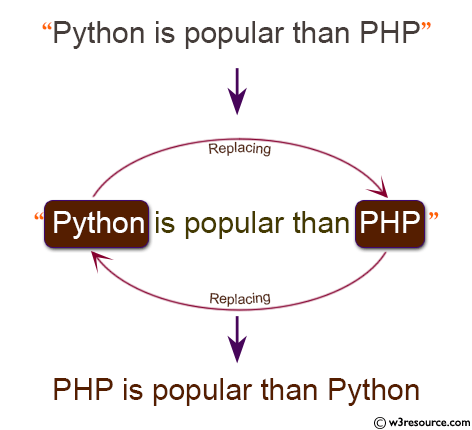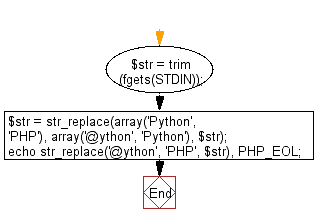﻿ PHP Exercise: Replace a string "Python" with "PHP" and "Python" with "PHP" in a given string - w3resource

# PHP Exercises: Replace a string "Python" with "PHP" and "Python" with "PHP" in a given string

## PHP: Exercise-63 with Solution

Write a PHP program to replace a string "Python" with "PHP" and "Python" with " PHP" in a given string.

Input: English letters (including single byte alphanumeric characters, blanks, symbols) are given on one line. The length of the input character string is 1000 or less.

Pictorial Presentation:Sample Solution: -

PHP Code:

``````<?php
\$str = trim(fgets(STDIN));
\$str = str_replace(array('Python', 'PHP'), array('@ython', 'Python'), \$str);
echo str_replace('@ython', 'PHP', \$str), PHP_EOL;

?>
```
```

Sample Input:
PHP is popular than Python

Sample Output:

`Python is popular than PHP.`

Flowchart:PHP Code Editor:

Have another way to solve this solution? Contribute your code (and comments) through Disqus.

What is the difficulty level of this exercise?

Test your Programming skills with w3resource's quiz.

﻿

## PHP: Tips of the Day

Mutates the original array to filter out the values specified

Example:

```<?php
function tips_pull(&\$items, ...\$params)
{
\$items = array_values(array_diff(\$items, \$params));
return \$items;
}

\$items = ['x', 'y', 'z', 'x', 'y', 'z'];
print_r(tips_pull(\$items, 'y', 'z'));
?>
```

Output:

```Array
(
 => x
 => x
)
```